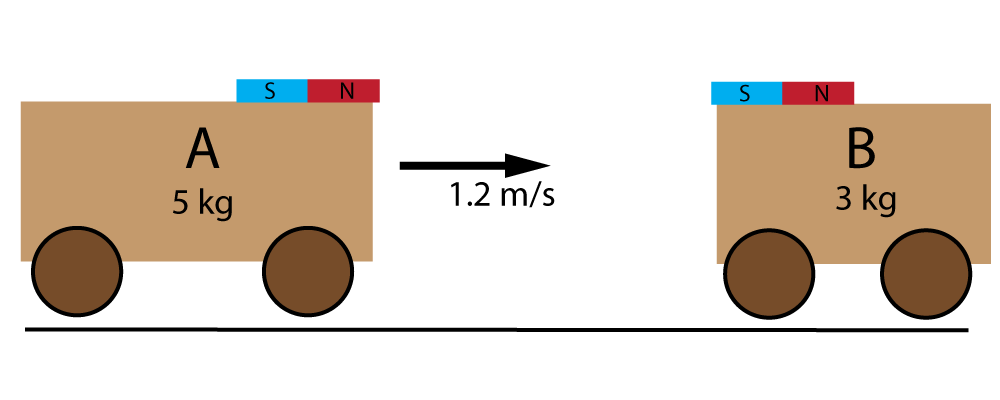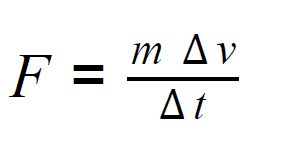µ GCSE | Momentum

10 quick questions - for GCSE and iGCSE

10 minutes maximum! (can you do it in 5?)

Grab a calculator - time for some maths!

(Mostly higher level stuff).

1. Which one of these is the correct formula for momentum?

• A. momentum = mass x acceleration
• B. momentum = mass ÷ acceleration
• C. momentum = mass x velocity
• D. momentum = mass ÷ velocity

What are the missing values in this table showing the momentum of different sports balls?mass velocity momentum 2. Basketball 1.5 kg 6 m/s ? 0.25 4 9 10 kg m/s 3. Tennis ball 200 g ? 400 100 0.4 10 m/s 2 kg m/s 4. Netball ? 48 19 5.33 0.19 kg 3 m/s 16 kg m/s

5. When 2 objects collide, they follow " the law of conservation of momentum" . This law states that..

• A. "The total momentum before and after a collision is the same if no external force acts on the system".
• B. "The total momentum before and after a collision is always the same".
• C. "The total momentum before and after a collision is always zero".
• D. "The total momentum of any system is always zero".

Q6-10.

To investigate this law, 2 'trolleys' (blocks of wood on wheels) were collided together. The trolleys have magnets on top so they stick together after the collision.

Trolley A is moving at 1.2 m/s towards B, which is stationary.6. What is the momentum of trolley A before the collsion?

• A. 2.5 kg m/s
• B. 5.0 kg m/s
• C. 6.0 kg m/s
• D. 9.6 kg m/s

7. What is the total momentum of both trolleys after the collision once they are stuck together?

• A. 3.0 kg m/s
• B. 3.6 kg m/s
• C. 6.0 kg m/s
• D. 9.6 kg m/s

8. What is the speed of both A+B (stuck together) after the collision?

• A. 0.75 m/s
• B. 1.2 m/s
• C. 3.0 m/s
• D. 6.0 m/s

The formula here (in two different forms) can be used to find the force needed to change the momentum of a system.9. If trolley A was pushed for 0.5 seconds to get it moving to 1.2 m/s at the start, how much force was applied?

• A. 0.6 N
• B. 2.4 N
• C. 6 N
• D. 12 N

10. During the impact, trolley A pushes on B, so trolley B pushes back on A.

This is a simple example of....

• A. Newton's First Law.
• B. Newton's Second Law.
• C. Newton's Third Law.
• D. Newton's Fourth Law.